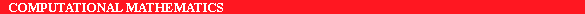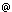ERCIM News No.22 - July 1995 - INRIA

# A Domain Decomposition Method

by Marina Vidrascu

The solution of elliptic problems using domain decomposition techniques has been of great interest in recent years because it produces parallel algorithms which efficiently solve linear systems arising from the finite element discretization of large problems in structural mechanics. Our objective is to be able to handle a large number of irregular unstructured domains using different types of finite elements. In order to obtain a reliable algorithm several obstacles must be overcome. In particular one must use an efficient preconditioner to solve iteratively the interface problem and develop robust automatic mesh partitioning algorithms.

Domain decomposition methods have been studied extensively in the past few years. The increasing success of these methods is due to the fact that they provide a high level of concurrenxcy and are simple to implement on most modern parallel computers. At INRIA, we have studied and implemented such algorithms within the Modulef and Menusin projects. The purpose of this paper is to briefly describe these research activities.

Readers coming recently to the field can find a complete bibliography in the Proceedings of the International Conferences on Domain Decomposition Methods which have been organized over the last eight years. The algorithms presented here below are described in details in the review article by Patrick Le Tallec "Domain decomposition methods in computational mechanics" (Computational Mechanics Advances 2, 1994).

Our objective is the efficient solution on parallel machines, using finite element methods, of problems in Computational Mechanics set on complex geometries and approximated on very fine grids. The linear system obtained after discretization is often very ill conditioned. The computation of the stiffness matrix and the solution of the system by a direct method is then extremely time and memory consuming. The development of effective parallel algorithms for solving this problem is a challenge. Domain decomposition methods, which mix local direct solvers and a global iterative interface solver turn out to be a very good answer to this challenge.

The principle of the method is to split the original domain of computation in smaller simpler subdomains, compute local simplified solutions, and use efficient iterative algebraic solvers to properly interface these solutions. The local interface domain decomposition algorithms are consistent from the mechanical point of view and can be written indifferently in terms of matrices or in terms of partial differential equations. Such algorithms involve, at each step and on each subdomain, the simultaneous solution of a displacement and of a traction problem. In addition, a coarse global solver of small size (6 x number of subdomains) has to be introduced to coordinate the rigid body motions of the different subdomains when solving equilibrium problems on a large number of subdomains,

The algorithm obtained is very efficient because it has both properties of numerical and parallel scalability. The numerical scalability means that the number of iterations of the iterative procedure does not grow or grows weakly with the number of subdomains or the mesh size.

On the other hand, a good domain decomposition algorithm cannot be efficiently used in an industrial environment without an automatic mesh partitioning algorithm. Defining the optimal criteria to split a domain in subdomains is not a trivial problem because it involves both numerical constraints (shape of the domains) and parallel implementation constraints (minimization of communications between processors, task balancing). The objective of partitioning is to split the problem into well balanced subdomains with minimal interaction and good aspect ratio. The algorithm developed is based on K means techniques previously developed in automatic data classification.

Both the domain decomposition and partitioning algorithms have been implemented (by Eric Saltel and Marina Vidrascu) in the finite element library Modulef. Parallel implementations (using directives) on shared memory parallel computers as the Cray C90 from I.D.R.I.S or the KSR1 are available. Recently a distributed memory version of the algorithm was implemented using PVM to preserve the portability, one of the important issues of the Modulef software.

Present work is particularly focussed on nonlinear elasticity. The approach used is to locally linearize the nonlinear equation via a Newton-like algorithm and then to solve the resulting linearized problems at each nonlinear iteration by a domain decomposition method. The domain decomposition algorithm and the automatic mesh partitioning have been succesfully used to solve real life complex three-dimensional structural problems.

E-mail : Marina.Vidrascuinria.fr